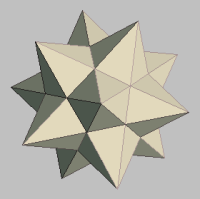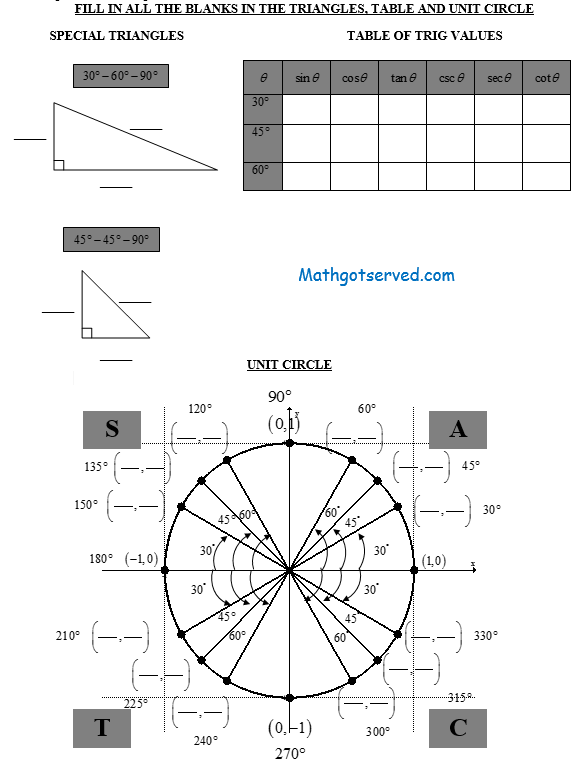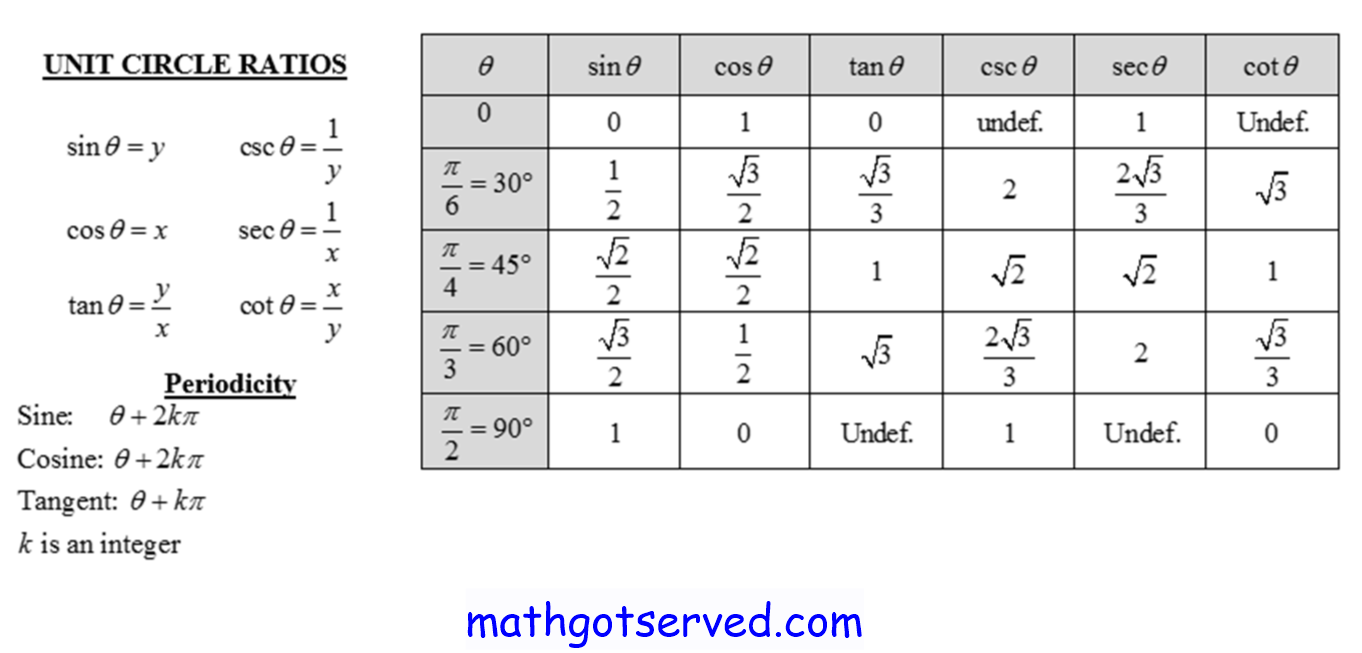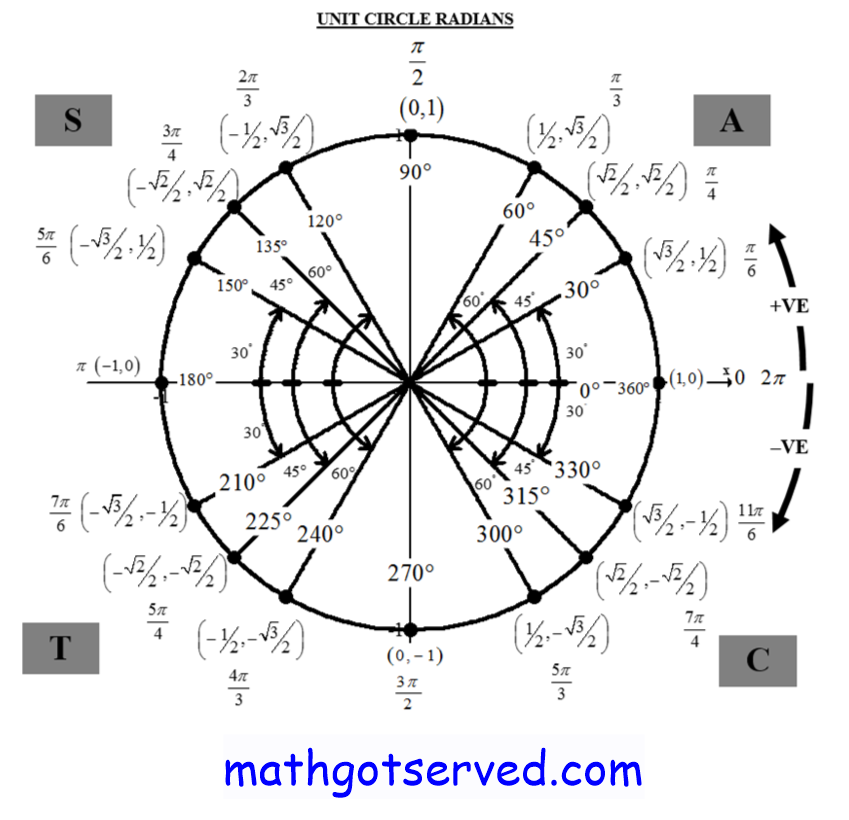MATHGOTSERVEDAlgebra 2 Common Core Functions: Transformations Operations and Inverses
TRANSFORMATION OF FUNCTIONS
Notes
The transformation of functions involves altering the size, position, or orientation of a function. The types of transformations that we will be considering in this section are 1) Dilations 2) Reflections 3) Shifts also known as translations.
Video Tutorial
Worked Out Examples on Transformation of Functions
The Transformation FormulaFINDING INVERSES
Meaning of Inverse: An inverse of a function f written as f-1 is the function the has the opposite effect of what the function f has. If the function f is viewed as a do function then the inverse can be viewed as the undo function the undoes what f does.
Strategy(Steps) How to Find the Inverse
Strategy(Steps) How to Find the Inverse
1. Numerical Method: Interchange the x and the y's to find the inverse of a functions. Note the inverse is not always a function. If the function passes the horizontal line test in order for it to have an inverse that is a function.
2. Graphical Method: Reflect the function along the line y=x
3.Switch the input with the inverse of the output and the output with the input and solve for the inverse of the output (If y as a function of x, switch x and y and solve)
Worked Out Examples
Video Tutorials
COMPOSITION OF FUNCTIONS
1.2.3.